chp5-5.mw

Let us look at an example of a market with three securites one of which is a risk-free security. Assume that the rates of return of the securites are given by the processes.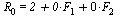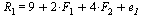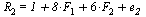Recall that the risk profile of a security in this market is the vector of the coefficents of the factors . Consequently, it is clear that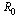is the risk-free rate since it has no risks - the coeffecients of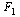and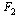in the representation of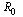is 0. Thus the risk profile ofis the vector (0,0).

Assume also that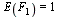,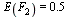,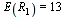and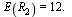Hence to confirm that, indeed, the expected  value of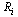i=1,...,2 is consisent with the numerical values above we execute the following:

For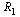we need to confirm that the equation below is correct

 > is(13=9+2*1+4*0.5);and  for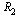we need to confirm the equation below is correct:

 > is(12=1+8*1+6*0.5);Note that we omit thebased on the APT assumption of  large portfolios  for which the averge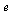will be zero, even though our example deals with only two securities.

The risk profile of security one is (2,4) and of security 2 is (8,6).  The optimization  problem which maximizes arbitrage profit is solved by Maple below, where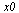stands for the proportion invested in the risk-free security.

 > simplex[maximize](x1*13+x2*12+2*x0,{x1+x2+x0=0,2*x1+8*x2=0,4*x1+6*x2=0});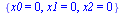Since the optimal solution to the maximization problem is the vector 0, it means that there are no arbitrage opportunities in this market.  The set of equations that must be satisfied in the λ's (prices of risks) if there are no arbitrage opportunities as solved below. Note that each equation states the excess rate of return over the risk-free rate for each of the risky securities. Thus we have

λ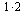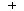λ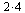=13-2, and

λ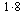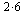=12-2

for the first and second securiyy respectively.

These equations are solved below.

 > solve({lambda0+lambda1*2+lambda2*4=13,lambda0+lambda1*8+lambda2*6=12,lambda0=2});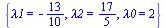>

Having solved for the price of risk we can find the expected rate of return that each security in this market should offer. For example, if in this market a security is introduced with a risk profile of  (3,3), its expected rate of return should be:

 > -3*13/10+3*17/5+2;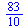> evalf(%);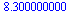Let us see if indeed when such a security is introduced to the market with the correct expected rate of return, no arbitrage oppurtunites are being intorduced. To this end, we solve the optimization probelm below.

 > simplex[maximize](2*x0+x1*13+x2*12+x3*83/10,{x0+x1+x2+x3=0,2*x1+8*x2+3*x3=0,\

 > 4*x1+6*x2+3*x3=0});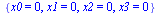>

Let's see what happens to the arbitrage maximization problem  if a security with a risk profile of (3,3) is introduced with an expected rate of retun of 84/10

 > simplex[maximize](2*x0+x1*13+x2*12+x3*84/10,{x0+x1+x2+x3=0,2*x1+8*x2+3*x3=0,\

 > 4*x1+6*x2+3*x3=0});

 >

This time the problem is unbounded, since arbitrage opportunities do exist, and thus MAPLE's output is NULL. In order to find an arbitrage portfolio we can change the problem as follows. Obviously, the problem was unbounded since unbounded positions were taken in at least some of the securites. Hence if we restrict the size of the positions we can have the problem bounded. As a result an optimal solution would exist and we can see the arbitrage portfolio.

Also in order to be able to read the expected rate of return of the arbitrage portfolio, we add a dummy varible ExpROR and  a dummy constrain; ExpROR=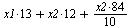. Thus the optimal vlaue of ExpROR will be the rate of return obtained by the arbitrage portfolio.

 > simplex[maximize](2*x0+x1*13+x2*12+x3*84/10,{x0+x1+x2+x3=0,2*x1+8*x2+3*x3=0,4*x1+6*x2+3*x3=0,ExpROR=x1*13+x2*12+x3*84/10,x0>=-1,x1>=-1,x2>=-1,x3>=-1});>

We have now  identified a (self-financed) arbitrage portfolio: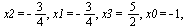with an expected rate of retrun of.

We therefore have demonstrated the use of the λ in determining the rate of return of a new security that is introduced to the market. We also have explained how to find, in a systematic way, an arbitrage portfolio if the market has arbitrage opportunities.